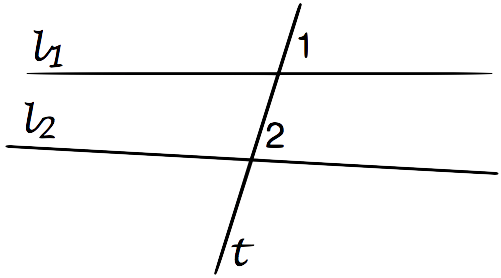The Geometry of y = mx + bHenri Picciotto

Most math students and teachers in the US never see a formal proof that the graph of y = mx + b is a straight line, and conversely that a non-vertical line satisfies an equation of the form y = mx + b.

The Common Core State Standards for 8th grade include this:

Use similar triangles to explain why the slope m is the same between any two distinct points on a non-vertical line in the coordinate plane; derive the equation y = mx for a line through the origin and the equation y = mx + b for a line intercepting the vertical axis at b.

In my view, 8th grade is a bit early for an airtight formal derivation, especially since at this stage students only have an informal understanding of similarity (according to the Common Core 8th grade standards!) Still, the idea of making that algebra-geometry connection at some point is a good one.

This worksheet, intended for teachers, guides the reader through such a derivation. (Prerequisite, obviously, is familiarity with both linear functions and similar triangles, as well as the maturity to appreciate the formal development of mathematical ideas.)

And this one goes on to prove a related result for function diagrams.Related pages on this site:
Teachers' Mathematics
Algebra
Geometry
Function Diagrams